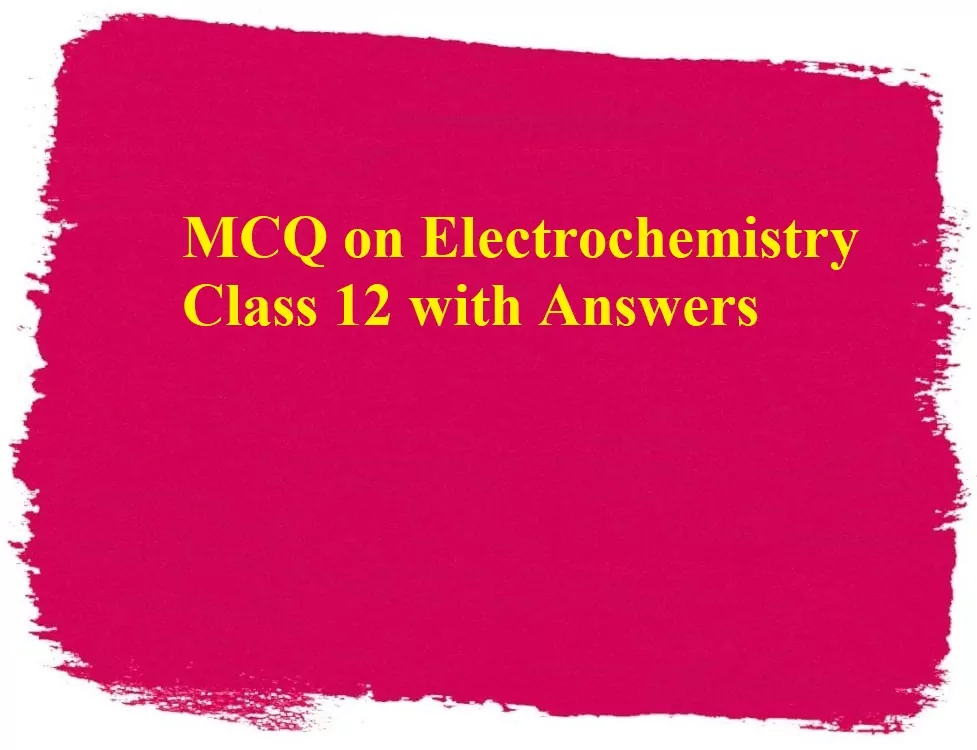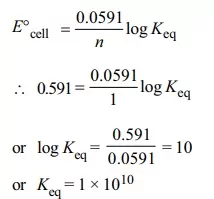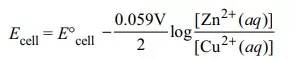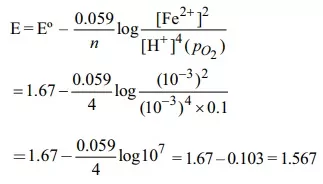# MCQ on Electrochemistry Class 12 with Answers# MCQ on Electrochemistry Class 12 with Answers

MCQ on Electrochemistry Class 12 with Answers

Que 1. When the sample of copper with zinc impurity is to be purified by electrolysis, the appropriate electrodes are
Cathode                                  Anode
(a) Pure zinc                            Pure copper
(b) Impure sample                  Pure copper
(c) Impure zinc                       Impure sample
(d) Pure copper                       Impure sample
Ans 1. (d)

Que 2. In the electrolytic cell, the flow of electrons is from
(A) Cathode to the anode in the solution
(B) Cathode to the anode through external supply
(C) Cathode to the anode through the internal supply
(D) Anode to the cathode through the internal supply
Ans 2. Cathode to the anode through the internal supply
Reason: In an electrolytic cell, cathode acts as a source of electrons.

MCQ on Electrochemistry Class 12 with Answers

Que 3. During the electrolysis of a solution of AgNO3, 96500 coulombs of charge pass through the electroplating both, the mass of silver deposited in the cathode will be
(A) 1.08 g                   (B) 10.8 g                    (C) 21.6 g                    (D) 108 g
Ans 3. (b)
Reason: Ag+ + e → Ag,
96500 C will liberate silver = 108 gm.
9650C will liberate silver = 10.8 g

Que 4. The standard emf of a cell, involving one electron change is found to be 0.591 V at 25°C. The equilibrium constant of the reaction is (F = 96500 C mol–1)
(a) 1.0 × 101                                        (b) 1.0 × 105
(c) 1.0 × 1010                                       (d) 1.0 ×1030
Ans 4. (c)
Reason: The E°cell is given byMCQ on Electrochemistry Class 12 with Answers

Que 5. For the galvanic cell Zn | Zn2+ (0.1M) || Cu2+ (1.0 M)|Cu the cell potential increase if:
(a) [Zn2+] is increased                                     (b) [Cu2+] is increased
(c) [Cu2+] is decreased                                    (d) surface area of anode is increased
Ans 5. (b)
Reason: For the given cellThe cell potential will decrease with increase in [Zn2+(aq)] and will increases with increase in [Cu2+(aq)]

Que 6. Consider the following cell reaction:
2Fe(s) + O2 (g) + 4H+ (aq) → 2Fe2+ (aq) + 2H2O(l)
E0 = 1.67V At [Fe2+] = 10–3 M, p(O2) = 0.1 atm and pH = 3, the cell potential at 25ºC is
(a) 1.47 V                                            (b) 1.77 V
(c) 1.87 V                                            (d) 1.57 V
Ans 6. (d)
Reason: Here n = 4, and [H+] = 10–3 (as pH = 3) Applying Nernst equationQue 7. The cell constant of a conductivity cell ___________.
(a) changes with the change of electrolyte.
(b) changes with the change of concentration of electrolyte.
(c) changes with the temperature of the electrolyte.
(d) remains constant for a cell.
Ans 7. (d)
Reason: Remains constant for a cell.

MCQ on Electrochemistry Class 12 with Answers

Que 8. Faraday’s law of electrolysis are related to the:
(a) Atomic number of the cation
(b) the atomic number of the anion
(c) the equivalent weight of the electrolyte
(d) speed of the cation
Ans 8. (c)
Reason: Faraday’s laws of electrolysis states that 96500 coulombs of charge is needed to deposit one gm equivalent of an element at the electrode. So, it is related to the equivalent mass of electrolyte.

Que 9. The charge required for the reduction of 1 mol of MnO4 to MnO2 is:
(a) 1 F                         (b) 3 F                         (c) 5 F                         (d) 4 F
Ans 9. (b)
Reason: MnO4 ​→ MnO2
The oxidation number of Mn in MnO4,

⇒x=7
The oxidation number of Mn in MnO2,
⇒x−4=0

∴ Change in
Hence, the charge required will be .

Que 10. Electrolysis of aq. CuSO4 produces:
(a) an increase in pH
(b) a decrease in pH
(c) either decrease or increase
(d) H2SO4 in the solution
Ans 10. (b)
Reason: After the electrolysis i.e. the process in which electric current is passed through an aqueous solution of some compound or elements which leads to breakdown into anions and cations.
The copper sulphate solution will break down into Cu2+ cation and SO4−2​ anion.
As a result, the solution of copper sulphate which is blue in colour will no longer retain. This SO4−2​ combines with H2​ and increases the acidity.

MCQ on Electrochemistry Class 12 with Answers